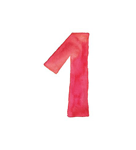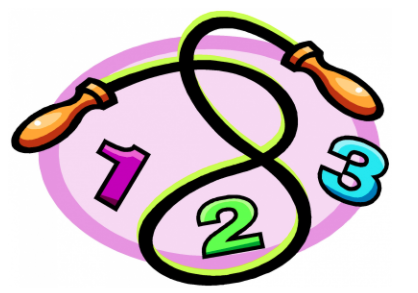# Skip Counting

Empowered Learner, Computational Thinker### Overview​

Skip counting is counting while skipping a number or numbers in between. To skip count the same count needs to be made every time. If counting by 3's you count every third number each time: 3,6,9,12,15. Skip counting can include counting either forwards or backward by a number other than 1. It is also a form of early multiplication.

Students will use Toy Theater's Number Charts to practice their skip counting.

Students will:

• Be able to skip count using various digits with the appropriate number chart.

Vocabulary Words:

1. Odd Numbers: Odd numbers are numbers that cannot be divided by two.

2. Even Numbers: Even numbers are a number that can be divided by two.

3. Skip Counting: Skip counting is the method of counting forward by numbers other than one.

4. Add: To add is to join (something) to something else so as to increase the size, number, or amount.

5. Repeated Addition: Repeated Addition is the process of repeatedly adding the same number, a strategy for introducing multiplication.

6. Subtract: To subtract is to take away (a number or amount) from another to calculate the difference.

7. Repeated Subtraction: Repeated Subtraction is a method of subtracting an equal number of items from a larger group. It is also known as division.

To prepare for this lesson:

• The teacher should make sure students are familiar with numbers and counting.

• The teacher should be familiar with the skip counting mats and how to move the chips.

• The teacher should introduce the website to students prior to the activity.

• Preview the video Skip Counting.

• Preview ABCYA's Skip Counting Game

• Preview Education.com's Game Skip-Counting

See Accommodations Page and Charts on the 21things4students.net site in the Teacher Resources.

Directions for this activity:

1. Introduce the students to skip counting by showing the videos.

2. Introduce the students to the skip counting mats.

3. The teacher should help the student select the appropriate mat for the student’s level.

4. The teacher should guide the student to skip count by giving the number to start with.

5. The student will drag the chip to cover the appropriate digit to follow the skip counting pattern.

6. Higher grade levels can give a multiplication equation.

7. The students can play computer games from ABCYA and Education.com.

Different options for assessing the students:

• Observations
• Check for understanding
• Exit tickets with number charts prepared for students.
• Class discussion of math talks, number talks.
• Create an assessment within the Learning Management System.
• Create FlipGrids

MITECS: Michigan adopted the "ISTE Standards for Students" called MITECS (Michigan Integrated Technology Competencies for Students) in 2018.

Empowered Learner
1a. Students articulate and set personal learning goals, develop strategies leveraging technology to achieve them and reflect on the learning process itself to improve learning outcomes.
1b. Students build networks and customize their learning environments in ways that support the learning process.
1c. Students use technology to seek feedback that informs and improves their practice and to demonstrate their learning in a variety of ways.
1d. Students understand the fundamental concepts of technology operations, demonstrate the ability to choose, use and troubleshoot current technologies and are able to transfer their knowledge

Computational Thinker
5a. Students formulate problem definitions suited for technology assisted methods such as data analysis, abstract models and algorithmic thinking in exploring and finding solutions.
5c. Students break problems into component parts, extract key information, and develop descriptive models to understand complex systems or facilitate problem-solving.
5d. Students understand how automation works and use algorithmic thinking to develop a sequence of steps to create and test automated solutions.

##### CONTENT AREA RESOURCES

Write a set of directions on how to skip count with a number chart. Lower level students can do this with an upper grade peer helper.

• Students learn Skip Counting Songs.
• Students create an illustration to show skip counting with an addition equation.

Create a math learning log page with number charts and skip counting patterns. The class has number talks and math talks on skip counting and repeated addition/subtraction..

CREDITS
This task card was created by Julie Hoehing, Lake Shore Public Schools, May 2021.